Select Page

# Deterministic Finite Automaton (DFA)

The finite automata are also called deterministic finite automata or automaton where Deterministic means uniqueness of the computation.

• DFA accept all finite regular languages and some infinite regular languages as well.
• For each sigma value (0, 1…n) there must be a provided path from each state.
• DFA cannot move to other state without any input character, so, Null move not accepted.
• DFA may have multiple final states.

A simple example of DFA is given below.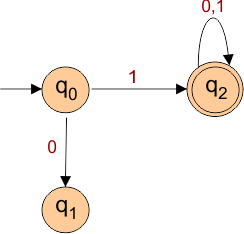## Formal Definition of a DFA

A DFA can be represented by a 5 tuples (Q, ∑, δ, q0, F) where

• Qis a finite set of all states (q0, q1, q2,   … qn). where n is finite number
• is a finite set of symbols called the alphabet. i.e. {0, 1,a,b},
• δis the transition function where δ: Q × ∑ → Q
• q0is the initial state from where any input is processed (q0 ∈ Q).
• Fis a set of final state/states where F will be subset ( ⊆ ) of Q.

## Graphical Representation of DFA

Graphical Representation of DFA is called state diagram

• The vertices represent the states.
• The arrow (à) labeled with an input alphabet () show the transitions (δ).
• The initial state (q0) is denoted by a circle with single incoming arrow.
• The final state is denoted by double circles.

Following figure shows all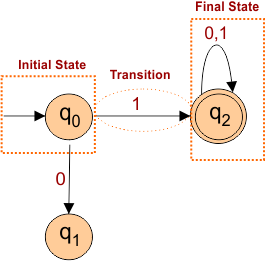## Construction of DFA

Suppose we are going to construct a DFA which accept a language of all strings, end with “a” and input sigma (∑) = {0, 1}. Then,

1. First draw all possible strings of that language.

L= {a, aa, aaa, ba, baba, aba, bbbba……….}

Note: epsilon and those strings which are ending with b (i.e. aabab) are not accepted.

2. For each sigma value (0, 1…n) there must be a provided path from each state (q0, q1, q2….qn).

Possibilities of path for each input from a any state

• May Go to next state if exist
• Self-loop
• Go to trap state
• May Go to previous state if exist

3. Now Draw the Automata model which will always according to generated language. Automata machine must accept the strings of given language (i.e. minimum length string to last maximum string).,

### Steps for Construction of DFA

Let construct DFA for language L= {a, aa, aaa, ba, baba, aba, bbbba……….}

Step 01: As Minimum length string is “a” which will be accepted as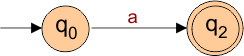Step 02: At initial state (q0), the path for input “a” is provided.  There must be a path for input “b” from initial state (q0).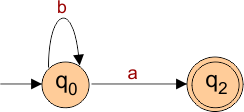Step 03: Now in the same way q1 must tell the path against each input  value of sigma “a” and “b” .  So final DFA which will accept all the strings of given language is given below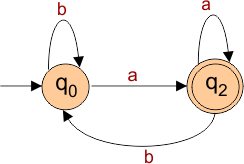## 5-Tuples of DFA

When DFA is constructed we can explain its 5-tuples easily. As the above DFA is explained below with 5-tuples

• Q = {q0, q1}   //states will be q0 and q1
• ∑ = {a, b}      // input already given in question
• q0 = {q0}        // start state is q0
• F = {q1}        // final state will become q1
• Transition function δ (δ: Q × ∑ → Q)as shown by the following table

As q0,q1 are states and a,b are input value of Sigma.

 Current State Next State for Input “a” Next State for Input “b” q0 q1 q0 q1 q1 q0

### Don’t Confuse

• If state names are a,b,c,d….z then  sigma values will be 0,1,2,3…n
• If state names are q0, q1, q2, q3…qn then sigma values can be a,b,c…z or 0,1,2,3…n

Note: state and sigma value cannot be same in notation as state “a” and sigma value “a” is not possible.

Help Other’s By Sharing…